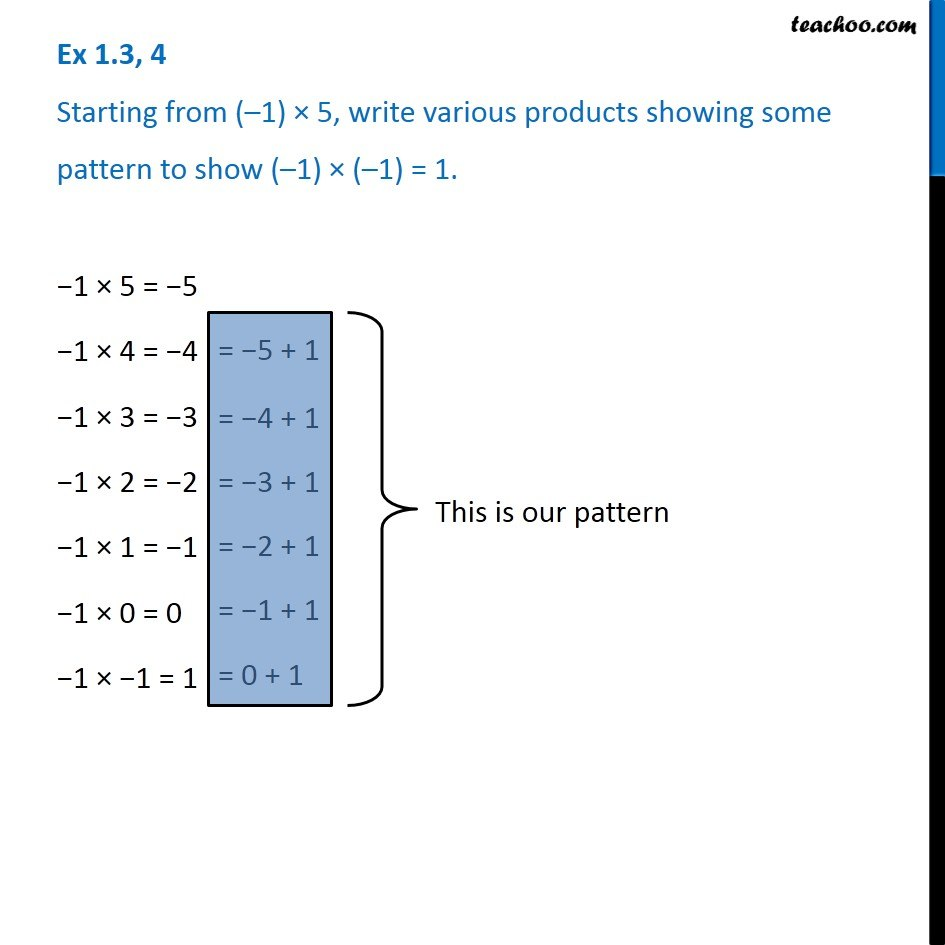1. Chapter 1 Class 7 Integers
2. Concept wise
3. Multiplication of Integers

Transcript

Ex 1.3, 4 Starting from (–1) × 5, write various products showing some pattern to show (–1) × (–1) = 1. −1 × 5 = −5= −5 + 1 −1 × 4 = −4= −4 + 1 −1 × 3 = −3= −3 + 1 −1 × 2 = −2= −2 + 1 −1 × 1 = −= −1 + 1 −1 × 0 = 0 −1 × −1 = 1= 0 + 1 This is our pattern